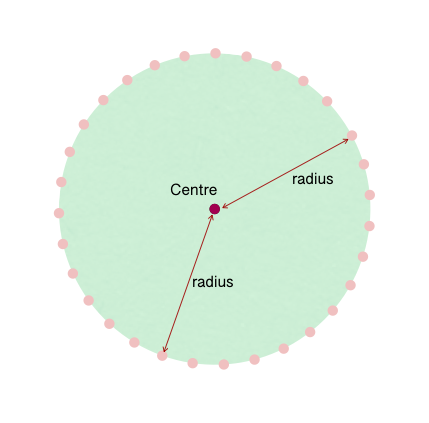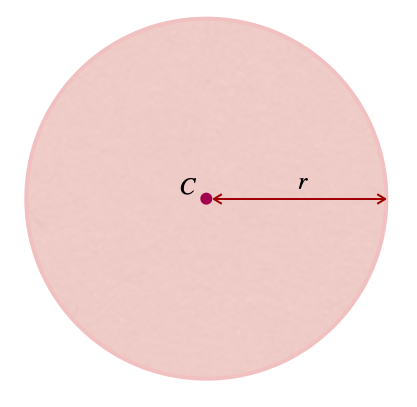# 7th Grade - Area of Circles

## Introduction

• A circle is a two-dimensional closed figure formed by joining a set of all the points equidistant from a fixed point.
• The fixed point is called the circle's center, and the fixed distance is called the circle's radius.• The most extended chord that passes through the center of a circle joining the two points on the circumference of a circle, is called its diameter.

$d=2r$, where $d=$diameter of the circle & $r=$radius of the circle.

• The circumference of a circle is the length of the boundary of a circle, and can be calculated using the formula $C=2\mathrm{\pi r}$, where $r=$radius of the circle.

## Area of Circles

• The area of a circle is the measure of the region enclosed inside it.
• The area of a circle depends on the length of its radius.
• If we know the radius of a circle, we can calculate its area using the formula mentioned below.$Are{a}_{circle}=\mathrm{\pi }·{\mathrm{r}}^{2}$, where $\mathrm{\pi }=\frac{22}{7}\approx 3.14$, and $r=$the radius of the circle.

• Likewise, if the diameter of a circle is known, its area can be calculated using the formula mentioned below.

$Are{a}_{circle}=\frac{\mathrm{\pi }}{4}·{\mathrm{d}}^{2}$, where $d=$the diameter of the circle $\left(d=2r\right)$

## Solved Examples

Example 1: What is the area of a circle with a radius of 7 cm?

Solution: $=\frac{22}{7}×{\left(7\right)}^{2}$$=22×7$

Example 2: What is the area of a circle with a diameter of 2 m? (Use $\mathrm{\pi }=3.14$)

Solution: $Are{a}_{circle}=\frac{\mathrm{\pi }}{4}·{d}^{2}$$=\frac{3.14}{4}×{\left(2\right)}^{2}$

Example 3: Find the circumference of a circle with a radius of .

Solution: $C=2\mathrm{\pi r}$$=2×\frac{22}{7}×\left(3\frac{1}{2}\right)$$=2×\frac{22}{7}×\frac{7}{2}$

## Cheat Sheet

• Relation between the diameter & radius of a circle: $d=2r$
• Circumference of a circle: $C=2\mathrm{\pi r}$
• Area of a circle when the radius is known: $Are{a}_{circle}=\mathrm{\pi }·{\mathrm{r}}^{2}$
• Area of a circle when the diameter is known: $Are{a}_{circle}=\frac{\mathrm{\pi }}{4}·{\mathrm{d}}^{2}$

## Blunder Areas

• The radius is half of the diameter, not the vice-versa.
• The area of a semi-circle is half the area of a circle.
• The perimeter of a semi-circle is not half the perimeter of a circle.